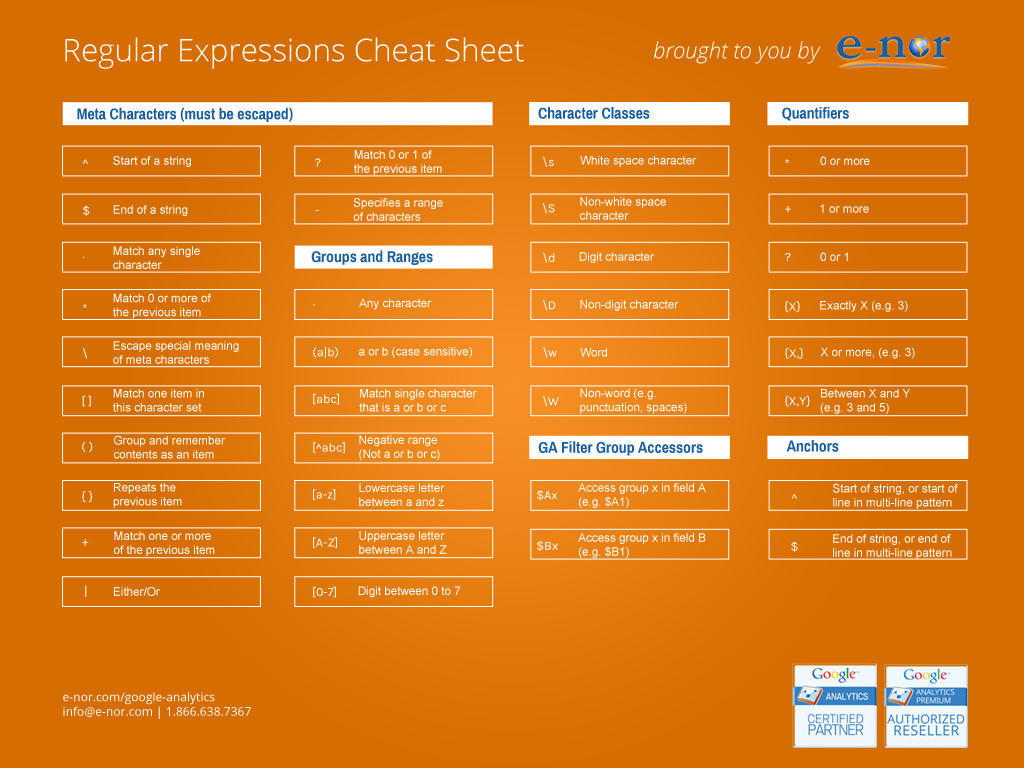``````var reg = /^(\d{1,4})(-|\/)(\d{1,2})\2(\d{1,2})\$/;
var r = fieldValue.match(reg);

#### 1 . 校验密码强度

``^(?=.*\d)(?=.*[a-z])(?=.*[A-Z]).{8,10}\$``

#### 2. 校验中文

``^[\u4e00-\u9fa5]{0,}\$``

#### 3. 由数字、26个英文字母或下划线组成的字符串

``^\w+\$``

#### 4. 校验E-Mail 地址

``[\w!#\$%&'*+/=?^_`{|}~-]+(?:\.[\w!#\$%&'*+/=?^_`{|}~-]+)*@(?:[\w](?:[\w-]*[\w])?\.)+[\w](?:[\w-]*[\w])?``

#### 5. 校验身份证号码

15位：

``^[1-9]\d{7}((0\d)|(1[0-2]))(([0|1|2]\d)|3[0-1])\d{3}\$``

18位：

``^[1-9]\d{5}[1-9]\d{3}((0\d)|(1[0-2]))(([0|1|2]\d)|3[0-1])\d{3}([0-9]|X)\$``

#### 6. 校验日期

“yyyy-mm-dd“ 格式的日期校验，已考虑平闰年。

``^(?:(?!0000)[0-9]{4}-(?:(?:0[1-9]|1[0-2])-(?:0[1-9]|1[0-9]|2[0-8])|(?:0[13-9]|1[0-2])-(?:29|30)|(?:0|1)-31)|(?:[0-9]{2}(?:0||)|(?:0||)00)-02-29)\$``

#### 7. 校验金额

``^[0-9]+(.[0-9]{2})?\$``

#### 8. 校验手机号

``^(13[0-9]|14[5|7]|15[0|1|2|3|5|6|7|8|9]|18[0|1|2|3|5|6|7|8|9])\d{8}\$``

#### 9. 判断IE的版本

IE目前还没被完全取代，很多页面还是需要做版本兼容，下面是IE版本检查的表达式。

``^.*MSIE [5-8](?:\.[0-9]+)?(?!.*Trident\/[5-9]\.0).*\$``

#### 10. 校验IP-v4地址

IP4 正则语句。

``\b(?:(?:25[0-5]|2[0-4][0-9]|?[0-9][0-9]?)\.){3}(?:25[0-5]|2[0-4][0-9]|?[0-9][0-9]?)\b``

#### 11. 校验IP-v6地址

IP6 正则语句。

``(([0-9a-fA-F]{1,4}:){7,7}[0-9a-fA-F]{1,4}|([0-9a-fA-F]{1,4}:){1,7}:|([0-9a-fA-F]{1,4}:){1,6}:[0-9a-fA-F]{1,4}|([0-9a-fA-F]{1,4}:){1,5}(:[0-9a-fA-F]{1,4}){1,2}|([0-9a-fA-F]{1,4}:){1,4}(:[0-9a-fA-F]{1,4}){1,3}|([0-9a-fA-F]{1,4}:){1,3}(:[0-9a-fA-F]{1,4}){1,4}|([0-9a-fA-F]{1,4}:){1,2}(:[0-9a-fA-F]{1,4}){1,5}|[0-9a-fA-F]{1,4}:((:[0-9a-fA-F]{1,4}){1,6})|:((:[0-9a-fA-F]{1,4}){1,7}|:)|fe80:(:[0-9a-fA-F]{0,4}){0,4}%[0-9a-zA-Z]{1,}|::(ffff(:0{1,4}){0,1}:){0,1}((25[0-5]|(2[0-4]|1{0,1}[0-9]){0,1}[0-9])\.){3,3}(25[0-5]|(2[0-4]|1{0,1}[0-9]){0,1}[0-9])|([0-9a-fA-F]{1,4}:){1,4}:((25[0-5]|(2[0-4]|1{0,1}[0-9]){0,1}[0-9])\.){3,3}(25[0-5]|(2[0-4]|1{0,1}[0-9]){0,1}[0-9]))``

#### 12. 检查URL的前缀

``````if (!s.match(/^[a-zA-Z]+:\/\//))
{
s = 'http://' + s;
}``````

#### 13. 提取URL链接

``^(f|ht){1}(tp|tps):\/\/([\w-]+\.)+[\w-]+(\/[\w- ./?%&=]*)?``

#### 14. 文件路径及扩展名校验

``^([a-zA-Z]\:|\\)\\([^\\]+\\)*[^\/:*?"<>|]+\.txt(l)?\$``

#### 15. 提取Color Hex Codes

``^#([A-Fa-f0-9]{6}|[A-Fa-f0-9]{3})\$``

#### 16. 提取网页图片

``\< *[img][^\\>]*[src] *= *[\"\']{0,1}([^\"\'\ >]*)``

#### 17. 提取页面超链接

``(<a\s*(?!.*\brel=)[^>]*)(href="https?:\/\/)((?!(?:(?:www\.)?'.implode('|(?:www\.)?', \$follow_list).'))[^"]+)"((?!.*\brel=)[^>]*)(?:[^>]*)>``

#### 18. 查找CSS属性

``^\s*[a-zA-Z\-]+\s*[:]{1}\s[a-zA-Z0-9\s.#]+[;]{1}``

#### 19. 抽取注释

``<!--(.*?)-->``

#### 20. 匹配HTML标签

``<\/?\w+((\s+\w+(\s*=\s*(?:".*?"|'.*?'|[\^'">\s]+))?)+\s*|\s*)\/?>``

#### 正则表达式的相关语法#### 学习正则表达式#### 正则表达式在线测试工具

regex101 是一个非常不错的正则表达式在线测试工具，你可以直接在线测试你的正则表达式哦。「技多不压身的设计师才有高薪资！」【优设网 原创文章 投稿邮箱：2650232288@qq.com】

================关于优设网================
“优设网uisdc.com“是国内人气最高的网页设计师学习平台，专注分享网页设计、无线端设计以及PS教程。
【特色推荐】评论 收藏